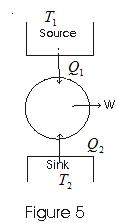# Thermodynamics

## 8. Work done in Isothermal process

• In an isothermal process temperature remains constant.
• Consider pressure and volume of ideal gas changes from (P1, V1) to (P2, V2) then, from first law of thermodynamics
ΔW = PΔV
Now taking ΔV aproaching zero i.e. ΔV𔾴 and suming ΔW over entire process we get total work done by gas so we have
W = ∫PdV
where limits of integration goes from V1 to V2
as PV = nRT we have P = nRT / V
W = ∫(nRT/V)dV
where limits of integration goes from V1 to V2
on integrating we get,
W=nRT ln(V2/V1)               (3)
Where n is number of moles in sample of gas taken.

### 9. Work done in an Adiabatic process

• For an adiabatic process of ideal gas equation we have
PVγ = K (Constant)               (14)
Where γ is the ratio of specific heat (ordinary or molar) at constant pressure and at constant voluume
γ = Cp/Cv
• Suppose in an adiabatic process pressure and volume of a sample of gas changs from (P1, V1) to (P2, V2) then we have
P1(V1)γ=P2(V2)γ=K
Thus, P = K/Vγ
• Work done by gas in this process is
W = ∫PdV
where limits of integration goes from V1 to V2
Putting for P=K/Vγ, and integrating we get,
W = (P1V1-P2V2)/(γ-1)          (16)
• In and adiabatic process if W>0 i.e., work is done by the gas then T2< T1
• If work is done on the gas (W<0) then T2 > T1 i.e., temperature of gas rises.

## 10. Heat Engine and efficiency

• Any device which convents heat continously into mechenical work is called a heat engine.
• For any heat engine there are three essential requirements.
(i) SOURCE : A hot body at fixed temperature T1 from which heat engine can draw heat
(ii) Sink : A cold body, at a fixed lower temprature T2, to which any amount of heat can be rijectd.
(iii) WOEKING SUBTANCE : The material, which on being supplied with heat will do mechanical work.
• In heat engine, working substances, could be gas in cylinder with a moving piston.
• In heat engine working substance takes heat from the sorce, convents a part of it into mechanical work, gives out rest to the sink and returns to the initial state. This series of operations constitutes a cycle.
• This cycle is represented in fig below• Work from heat engine can be continously obtained by performing same cycle again and again.
• Consider,
Q1 - heat absorbed by working substance from sorce
Q2 - heat rejected to the since
W - net amount of work done by working substance
Q1-Q2 - net amount of heat absorbed by working substance.
ΔU = 0 since in the cycle Working Substance returns to its initial condition.
So on application of first law of thermodynamics
Q1-Q2 = W
• Thermal efficiency of heat engine
η= work output in energy units / Heat input in same energy units
= W / Q1 = (Q1-Q2 )/ Q1
Or, η = 1-(Q2/Q1)                    (17)
from this equation it is clear that
Q = 1 for Q2=0
and there would be 100% conversion of heat absorbed into work but such ideal engines are not possible in practice.

link to this page by copying the following text